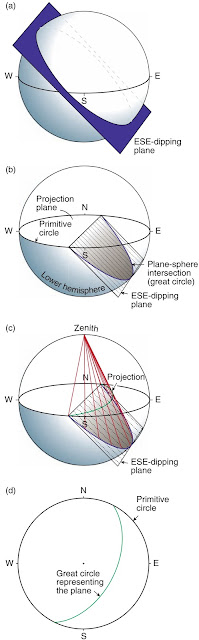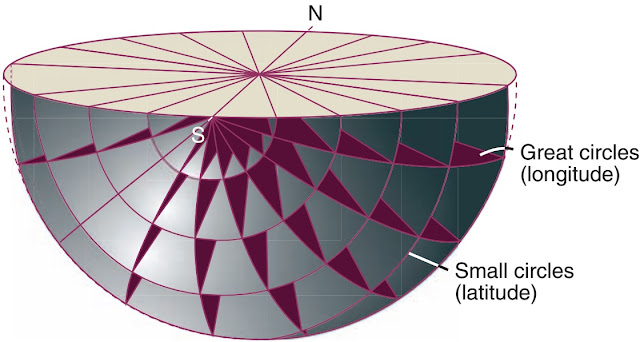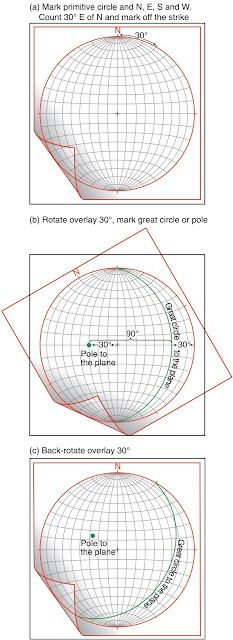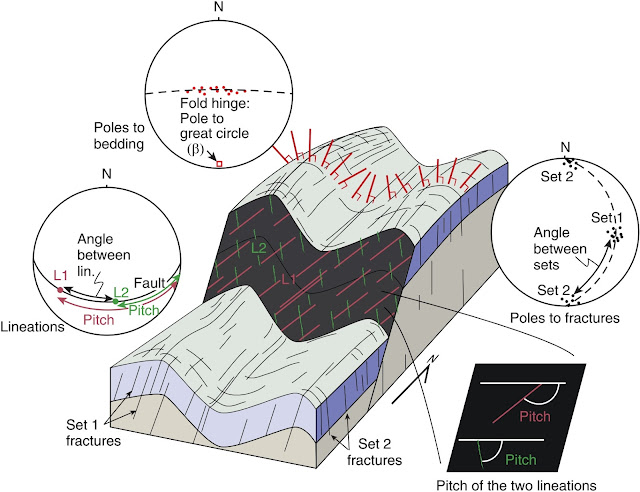### EquiangularFig. 1. Stereographic projection of an ESE-dipping plane.
Stereographic projection is about representing planar and linear features in a two-dimensional diagram. The orientation of a plane is represented by imagining the plane to pass through the centre of a sphere (Fig. 1a). The line of intersection between the plane and the sphere will then represent a circle, and this circle is formally known as a great circle. Except for the ﬁeld of crystallography, where upper-hemisphere projection is used, geologists use the lower part of the hemisphere for stereographic projections, as shown in Fig. 1b. We would like to project the plane onto the horizontal plane that runs through the centre of the sphere. Hence, this plane will be our projection plane, and it will intersect the sphere along a horizontal circle called the primitive circle
To perform the projection we connect points on the lower half of our great circle to the topmost point of the sphere or the zenith (red lines in Fig. 1c). A circleshaped projection (part of a circle) then occurs on our horizontal projection plane, and this projection is a stereographic projection of the plane. If the plane is horizontal it will coincide with the primitive circle, and if vertical it will be represented by a straight line. Stereographic projections of planes are formally called cyclographic traces, but are almost always referred to as great circles because of their close connection with great circles as deﬁned above.
Once we understand how the stereographic projection of a plane is done it also becomes obvious how lines are projected, because a line is just a subset of a plane. Lines thus project as points, while planes project as great circles. A great circle (as any circle) can be considered to consist of points, each of which represents a line withinthe plane. Hence, a line contained in a plane, such as a slickenline or mineral lineation, will therefore appear as a point on the great circle corresponding to that plane.Fig. 2. Stereographic projection of a line, in this case the normal or pole to a plane.
In Fig. 2 we have also projected the line that is normal to a given plane, represented by the pole to the plane. The projection is found by orienting the line through the center and connecting its intersection with the lower hemisphere with the zenith (red line in Fig. 2a). The intersection of this (red) line with the projection plane is the pole to the plane. Hence, planes can be represented in two ways, as great circle projections and as poles. Note that horizontal lines plot along the primitive circle (completely horizontal poles are represented by two opposite symbols) and vertical lines plot in the centre.Fig. 3. The stereonet. To compare this with longitude and latitudes of our planet, remember that the north–south axis is horizontal in the stereonet, and vertical in common views of the Earth.
For stereographic projections to be practical, we have to establish a grid of known surfaces for reference. We have already equipped the primitive circle with geographical directions (north, south, east and west), and we can compare the sphere with a globe with longitudes and latitudes. In three dimensions this is illustrated in Fig. 3, looking from the south pole toward the north pole: longitudes and latitudes are the lines of intersection between great circles (the original meaning) and so-called small circles. If we now project the small and great circles onto the horizontal projection plane, typically for every 2 and 10 degree interval, we will get what is called a stereographic net or stereonet.
The longitudes are planes that intersect in a common line (the N–S line), and thus appear as great circles in the stereonet. The projections of the latitudes, which are not planes but cones coaxial with the N–S line, are usually referred to as small circles (also their projections onto the stereonet). The net that emerges from the particular projection described above is called the Wulff net.

### Equal area projectionFig. 4. The equal area net.Fig. 5. The equal area projection. A plane is projected onto the projection plane, which in this case has been made tangential to the lower pole of the sphere. The projection is illustrated in 3-D (a) and along a proﬁle through C and A (b).Fig. 6. Two hundred and fifty quartz c-axes measured on the U-stage and plotted in the stereonet. An asymmetrical pattern with respect to the foliation (trending E–W in the plot), such as the one shown here, indicates the sense of shear.
The Wulff net makes it possible to work with angular relations (it preserves angles between planes across the net), which can be useful in some cases, for instance for crystallographic purposes. However, for most structural purposes it is more useful to preserve area, so that the densities of projections in one part of the plot can be directly compared to those of another. The method of plotting is the same, but because the projection is not stereographic but equal area (Fig. 4), the positions of planes and lines in the plot become somewhat different. The net is called a Schmidt net or simply an equal area net (Fig. 5 shows the equal area projection). Multiple data plotted in an equal area net can be contoured with respect to density, which can be useful when evaluating concentrations of structural data around certain geographic directions. Contouring is typically done for crystallographic axes such as quartz c-axes (Fig. 6), and the contour values exhibit the number of points as the percentage within a given 1% area of the stereonet. Contouring is easily done by means of one of the many computer programs available for personal computers.

### Plotting planes

Planes can be represented in a stereonet in two different ways: by means of great circles or poles (Fig 2). Fig. 5 gives a demonstration of how to plot both by hand, and we will start with a great circle representation.Fig. 7. Plotting the plane N 030 E, 30 NE in the stereonet (equal area projection).
A plane striking 030 (or N 30 E) and dipping 30 to the SE is plotted as an example. Tracing paper is placed over a pre-made net (an equal area net was chosen), and the centers are attached by means of a thumb tack. The primitive circle and north (N) are marked on the transparent overlay. We then mark off the strike value of our plane, which is 030 (Fig. 7a), and then rotate the overlay so that this mark occurs above the N direction of the underlying stereo plot (Fig. 7b). For our example, this involves rotating the overlay 30 anticlockwise. We then count the dip value from the primitive circle inwards, and trace the great circle that it falls on (Fig. 7b). When N on the tracing paper is rotated back to its original orientation (Fig. 7c) we have a great circle that represents our plane. The shallower its dip, the closer it comes to the primitive circle, which itself represents a horizontal plane.
The procedure is quite similar if we want to plot poles. All we do differently is to count the dip from the center of the plot in the direction opposite to that of the dip, which in our example is to the left. When done correctly, there will be 90 between the great circle and the pole of the same plane (see Fig. 7b). The pole thus falls on the opposite side of the diagram from that of the corresponding great circle. Poles are generally preferred in structural analyses that involve large amounts of orientation data, and particularly if grouping of structural orientations is an issue (which commonly is the case).

### Plotting linesFig. 8. Plotting the line plunging 40 degrees towards N 030 E in the stereonet (equal area projection).
Plotting a line orientation is similar (but different) to plotting a plane orientation. For example, a line plunging 40 degrees toward 030 (NE) is considered. As for the plane, we mark off the trend (030) (Fig. 8a), rotate the overlay either 30 degrees anticlockwise, as for the plane (Fig. 8b), or until it reaches the E direction (a clockwise rotation of 60 degrees for our example). Now count the plunge value along the straight line toward the center, starting at the primitive circle, and mark off the pole (Fig. 8b). Back-rotate the overlay, and the task is completed (Fig. 8c).

### Pitch (rake)Fig. 9. A constructed, but realistic, situation where various structural elements in a deformed rock sequence are represented in stereonets. Plotting bedding orientations reveals the b-axis (local orientation of the hinge line). The angle between the fracture sets can be found by counting degrees along the great circle that ﬁts both data sets. Fault data are plotted separately, showing the fault plane as a great circle and lineations as dots on that great circle.
When doing fault analyses it is useful to plot both the slip plane and its lineation(s) in the same plot. In this case the lineation will lie on the great circle that is representing the slip plane. The angle between the horizontal direction and the lineation is called the rake or pitch, and is plotted by rotating the great circle of the plane to a N–S orientation and then counting the number of degrees from the horizontal (N or S), i.e. the pitch value measured in the ﬁeld (Fig. 9). Users of the right hand rule will always measure the pitch clockwise from the strike value, so that the angle could be up to 180 degrees . The right-hand rule has been used in Fig. 9. Others measure the acute angle and count from the appropriate strike direction, in which case the pitch will not exceed 90 degrees.

### Fitting a plane to lines

If two or more lines are known to lie in a common plane, the plane is found by plotting the lines in a net. The lines are then rotated until they fall on a common great circle, which represents the plane we are looking for.

### Line of intersection

The line of intersection between two planes is perhaps most easily seen by plotting the great circles of the two planes, in which case the line of intersection is represented by the point where the two great circles cross. When plotting poles to planes, the line of intersection is the pole to the great circle that ﬁts (contains) the two poles.

### Angle between planes and lines

The angle between two planes is found by plotting the planes as poles and then rotating the tracing paper until the two points fall on a great circle. The angle between the planes is then found by counting the degrees between the two points on the great circle (Fig. 9, where the angle of two sets of fractures is considered). The angle between two lines is found in a similar manner, where the two lines are ﬁtted to a great circle (the plane containing both lines) and the distance between them (in degrees) represents the angle (Fig. 9, lineations).

### Orientation from apparent dips

Finding the orientation of a planar structure from observations of apparent dips can sometimes be useful. If two or more apparent dips are measured on two arbitrarily oriented planes, and each of those two planes is represented by a great circle and a point representing the apparent dip (measured at the outcrop), then the great circle that best ﬁts the points represents the plane we want to ﬁnd. An inverse problem would be to determine apparent dips of a known planar fabric or structure as exposed on selected surfaces. We then plot the planar fabric as a great circle, and the apparent dip will be deﬁned by the point of intersection between the planar fabric and the surface of interest.

### Rotation of planes and lines

Rotation of planar and linear structures can be done by moving them along a great circle, the pole of which represents the rotation axis. Rotation about a horizontal axis is easy: just rotate the tracing paper so that the rotation axis falls along the N–S direction, and then rotate poles by counting degrees along the small circles.
Rotating along an inclined axis is a bit more cumbersome. It involves rotating everything so that the axis of rotation becomes horizontal, then performing the rotation as above, and ﬁnally, back-rotating so that the axis of rotation achieves its original orientation.

### Rose diagramFig. 10. The plots show the variations within each subarea, portrayed by means of poles, rose diagrams, and an arrow indicating the average orientation. The number of data within each subarea is indicated by “n”.

Sometimes only the strike component of planes is measurable or of interest, in which case the data can be represented in the form of a rose diagram. A rose diagram is the principal circle subdivided into sectors, where the number of measurements recorded within each sector is represented by the length of the respective petal. This is a visually attractive way of representing the orientation of fractures and lineaments as they appear on the surface of the Earth, and can also be used to represent the trend distribution of linear structures (Fig. 10).

### Plotting programs

All of these operations and more can be done more quickly by means of stereographic plotting programs, such as the one generously made available to the structural community by Richard Allmendinger (1998). However, understanding the underlying principles is the key to success when using such programs. Several plotting programs also have statistical add-ons that are quite useful.

Credits: Haakon Fossen (Structural Geology)